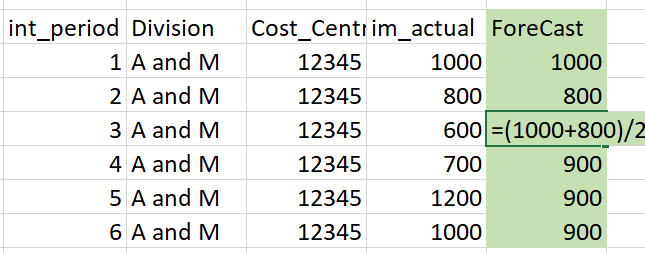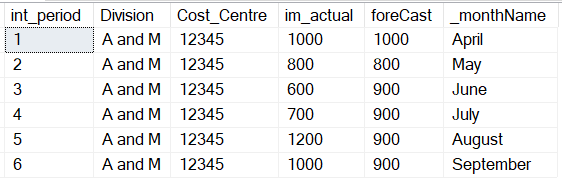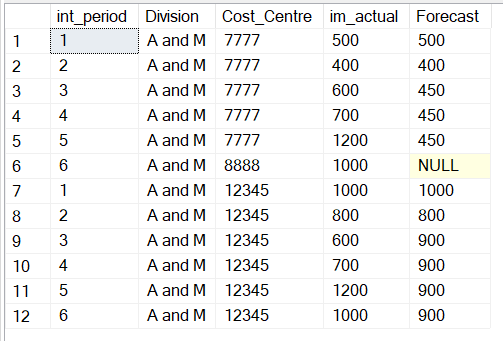# Run Total Forecast Recursive

Hi All
I would be grateful if you can help me to calculate the RunRate(forecast) on the following table .
The run rate will be calculate only on the future months on the field 'im_actual' , and the completed months (Past_Months) the value of 'Im_actual' will be same.

The field 'int_period' keep Fiscal months , so the completed month are , Apr(1) and May(2) where , June(3) onward are future month where we need to calcuatel ForeCast/RunRaate,
The completed month we don't need to calcuate and the 'Im_actual' values for completed will be same as exist in the table/field(im_actual) ,
To calculate the Forecast/RR for future months. it should sum of the completed months so in this case will be i.e. (1 and 2) and divide by 2(last completed month), so the calculate valurs for month (3 to 12) will be constant values.

Similarly, if I wants to calculate the Fore-cast after the month June(3) completed , the future Forecast will be sum of (month [1 to 3] and divided by 3 and this same/constact values will show for furtre moths 4 to 12..

The dummy data set script I've copied below

I've tried using recursive sql and UNION by split into two data set but not successfull

Thanks Again , if you have any question please feel free to ask me

Many thanks
Farhan

hi

is it possible to explain in simple terms .. i am finding it hard to understand !!!

it helps a lot if people can understand what you are saying easily and simply !!
may be its just me !!

doing the SQL is the easy part !!!
many senior experienced people are there in this forum ..

understanding the people who are asking the questions !! ..
is the only tough part !!!

hi hope this helps.. sorry

``````-------------------------------------------------------------------------
= calculate RunRate(forecast)

run rate calculated on  'im_actual'  = column
based               on  'int_period' = column (Fiscal months )
------------------------------------------------------------------------
-- My understanding

Forecast/RR = sum completed months (1 and 2) / 2(last completed month)
Forecast/RR = sum completed months (1 and 3) / 3(last completed month)
Forecast/RR = sum completed months (1 and 4) / 4(last completed month)
.
.
.
.
Forecast/RR = sum completed months (1 and 12) / 12(last completed month)
----------------------------------------------
- Your Statements .. what they mean ??

the value of  will be same.
and this same/constact values will show for furtre moths 4 to 12..
so the calculate valurs for month (3 to 12) will be constant values.
-----------------------------------------------
``````

Another idea is to show in excel with data .. and explanation !!!!!

hi

sum () over( rows preceding ) / last completed month

Sorry Hariss
Thanks for your response sorry my english is not the first langue so hard to explain.
The required result is attached in the Forecast field , you'll notice the completed month 1 and 2 , Apr and May have same value as "im_actual" i.e 1000 and 800 respectively, where the future months its simply sum of completed months and divided by 2 , and apply rest of all the future month i.e. (3 to 12).
But this example only apply for one cost-centre(12345) it should change based on each cost centre 'im_actual' value and calculate seperatly.
Hope make sense nowSo next one will be (600 + 700 + 1200)/3

?

Yes you're right the last completed month I can pass thorugh parameter so I'm not worry about that .
only thin is to calculate the 'future' month thinking to do using Recurse query in three differen part

Part1 = Simply show the "im_actual" = "Forecast" for complete month using < Last_Complete month
Part2 = Sum of 'im_actual" and assign into 'ForeCast' and use the 'xx' month to get one row sum(im_actual)/LastCompleted mont where <= Last Complete month

Part 2 = Replicate the Forecast value from 'Part2' result into for future the future months ?
Some how I can use CTE/Recurseive or UNION ALL query
Three different query (query 2 and query 3 will be joined ) to replicate constant value Sum(im_actual for april /may)/2 and pass into Forecast colum

Query 1
select im_actual = forecast from table where int_period <= completed month
Query 2
select sum(im_actual) = forecast from table where int_period <=completed month
Query 3 ( using join of query 2)
select sum(im_actual) = forecast from table where int_period > completed month

No next one will be constant value Forecast = 900 as shown in the example (in green color) as these
month are in future (3 to 12)
Required result shown below under 'Forecast' field:

What determines 1 to be April?

int_period =1 (April)
1 = April
2 = May
3 = June
4 = Jul and so on 12 = Mar

``````  WITH
cte_main AS
(

----Part Two (Calculate of Total for completed month)
SELECT
--'Caculate Total' as QueryNo
99 as int_period
, d1.Division
, d1.Cost_Centre
, 0 as im_actual
, sum(d1.im_actual) as ForeCast
FROM Forecast_Test d1
where d1.Cost_Centre=12345
and d1.int_period <=2
group by d1.Division, d1.Cost_Centre

UNION ALL

SELECT
-- 'Replicate Total for Futre Month ' as QueryNo
d2.int_period
,d2.Division
,d2.Cost_Centre
,d2.im_actual
,cte_main.Forecast  AS Forecast
FROM Forecast_Test d2
inner join cte_main on cte_main.Division = d2.Division and cte_main.cost_Centre = d2.Cost_Centre
where d2.Cost_Centre=12345
and d2.int_period > 2
--group by d1.Division, d1.Cost_Centre

)
--
--Part1 (Complete Month forecast same as actuals)
SELECT
--'Completed Mont ' as QueryNo
int_period
,Division
,Cost_Centre
,im_actual
,im_actual as ForeCast
FROM Forecast_Test
where Cost_Centre=12345
and int_period <2

UNION ALL
select * from cte_main``````

My SQL generate error

Msg 530, Level 16, State 1, Line 1
The statement terminated. The maximum recursion 100 has been exhausted before statement completion.

I dont think it will help if you keep posting and it muddies the already confusing question

Who determined 1 to be April, is this some non Gregorian calendar, Hijri calendar or some other calendar type or just your own business rule?

Sorry its the fiscal month (fiscal year ) start from April to March

Might this work?``````use sqlteam
go

create table fiscal_year(
_period int,
_month int,
_monthName varchar(50)
)

insert into fiscal_year
select distinct column_id,
from sys.all_columns
where column_id between 1 and 12
order by 1

create table
Forecast_Test
(
int_period integer,
Division VARCHAR(10),
Cost_Centre integer,
im_actual integer
);

INSERT INTO Forecast_Test values (1 , 'A and M', 12345, 1000)
INSERT INTO Forecast_Test values (2 , 'A and M', 12345, 800)
INSERT INTO Forecast_Test values (3 , 'A and M', 12345, 600)
INSERT INTO Forecast_Test values (4 , 'A and M', 12345, 700)
INSERT INTO Forecast_Test values (5 , 'A and M', 12345, 1200)
INSERT INTO Forecast_Test values (6 , 'A and M', 12345, 1000)
INSERT INTO Forecast_Test values (1 , 'A and M', 7777, 500)
INSERT INTO Forecast_Test values (2 , 'A and M', 7777, 400)
INSERT INTO Forecast_Test values (3 , 'A and M', 7777, 600)
INSERT INTO Forecast_Test values (4 , 'A and M', 7777, 700)
INSERT INTO Forecast_Test values (5 , 'A and M', 7777, 1200)

INSERT INTO Forecast_Test values (6 , 'A and M', 8888, 1000)

;with src
as
(
select AVG(im_actual) as foreCast, Cost_Centre
From Forecast_Test f
join fiscal_year fy on f.int_period = fy._period
where fy._month < month(getdate())
group by Cost_Centre
)
select f.int_period, f.Division, f.Cost_Centre,im_actual,
case
when fy._month < month(getdate()) then im_actual
--when fy._month > month(getdate()) then fc.foreCast
when fy._month >= month(getdate()) then src.foreCast
end as foreCast,
_monthName
From Forecast_Test f
join fiscal_year fy on f.int_period = fy._period
left join src on src.Cost_Centre = f.Cost_Centre
order by _month

drop table Forecast_Test
drop table fiscal_year
``````
``````declare @param int = 2

;WITH
cte_main AS
(
select * from Forecast_Test
)

select b.int_period,b.Division,b.Cost_Centre,b.im_actual
,case when b.int_period < = @param then b.im_actual else b.Forecast end as Forecast
from(
select a.*
,(
select avg(im_actual)  from cte_main where int_period <= @param and Division = a.Division and Cost_Centre = a.Cost_Centre
) as Forecast
from(
select * from cte_main
)a)b``````

hi Farhan

Looks like you got your solution ..

the way you explained in excel was NICE !!!
that was easy to understand !!! ...hi farhan

just for my .. practice i did this .. i wrote SQL which is different than yours
if it helps .. great``````declare @param int = 2

select   a.*
, case when int_period <= @param then a.im_actual else b.avg_ia end as Forecast
from
Forecast_Test a
left join
( select Cost_Centre,avg(im_actual) as avg_ia from Forecast_Test where int_period <= @param group by Cost_Centre ) b
on a.Cost_Centre= b.Cost_Centre
order by a.Cost_Centre, a.int_period
``````Thanks a lot
Really appreciate that . but I also need to cater the Division field in group by along with Cost center.
the calculate should look both Division+Cost Centre together sorry my example of 'Test' is not correct that's why i think you didn't consider that .

Kind regards and Thanks again Haris really appreciate that.
People like you keep this forum up and running
Farhan